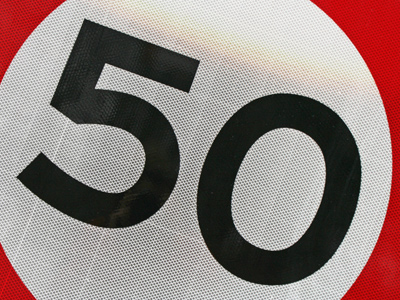10 x 5 = 50.

# 5 Times Table

This Math quiz is called '5 Times Table' and it has been written by teachers to help you if you are studying the subject at elementary school. Playing educational quizzes is an enjoyable way to learn if you are in the 3rd, 4th or 5th grade - aged 8 to 11.

It costs only \$12.50 per month to play this quiz and over 3,500 others that help you with your school work. You can subscribe on the page at Join Us

Learning the 5 times table is all about multiplying numbers by 5. This is one of the easier times tables to learn. One trick that might help you in learning the 5 times table is to multiply the numbers by 10 and then halve them. For example 4 x 5. If you multiply 4 x 10, you get 40. Half of 40 is 20. Therefore 4 x 5 must equal 20.

See how well you do in the following 5 times table quiz.

1.
9 x 5 =
14
25
40
45
To multiply a number by 9 first times it by 10 and then take it away. So 5 x 10 = 50 and 50 - 5 + 45
2.
6 x 5 =
11
30
35
60
3.
8 x 5 =
35
38
40
48
4.
7 x 5 =
35
40
42
45
7 x 5 is the same as (5 x 5) + (2 x 5). 5 x 5 = 25 and 2 x 5 = 10. 25 + 10 = 35 so 7 x 5 = 35
5.
12 x 5 =
55
59
60
65
To times any number by 5 just times it by 10 and then half it, so 12 x 10 = 120 and 120 ÷ 2 = 60
6.
5 x 5 =
10
15
20
25
When a number is multiplied by itself we say that it has been squared, so 5 squared is 25
7.
11 x 5 =
50
55
52
58
8.
4 x 5 =
9
12
16
20
4 x 5 can be either 4 + 4 + 4 + 4 + 4 or 5 + 5 + 5 + 5
9.
2 x 5 =
2
5
10
15
10.
3 x 5 =
10
12
15
18
Author:  Amanda Swift